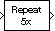# Repeat

Resample input at higher rate by repeating values

•Libraries:
DSP System Toolbox / Signal Operations
Communications Toolbox / Sequence Operations
DSP System Toolbox HDL Support / Signal Operations

## Description

The Repeat block upsamples each channel of the Mi-by-N input to a rate L times higher than the input sample rate. To do so, the block repeats each consecutive input sample L times at the output. Specify the integer L in the Repetition count, L parameter or through the input port L.

You can use the Repeat block in triggered subsystems when you set the Rate options parameter to ```Enforce single-rate processing```.

## Ports

### Input

expand all

Specify the input signal as a real or a complex-valued scalar, vector, or a matrix.

When you set Input processing to `Columns as channels (frame based)` and Rate options to ```Enforce single-rate processing```, the input can be a variable-size signal. When the input is a variable-size signal, the frame size (number of rows) and the number of channels (columns) of the signal can change during simulation.

This port is unnamed until you set Repetition count source to ```Input port```. (since R2023a)

Data Types: `single` | `double` | `int8` | `int16` | `int32` | `uint8` | `uint16` | `uint32` | `Boolean` | `fixed point`
Complex Number Support: Yes

Since R2023a

Specify the repetition count L as a positive integer less than or equal to the value you specify in the Maximum repetition count, Lmax parameter Lmax.

#### Dependency

To enable this port, set the Repetition count source parameter to ```Input port```.

Data Types: `single` | `double` | `int8` | `int16` | `int32` | `uint8` | `uint16` | `uint32`

### Output

expand all

The Repeat block outputs a signal that has the same data type and complexity as the input signal in. The size of the output depends on the value of the repetition count and the option you select in the Rate options parameter.

If you set Repetition count, L to L and Rate options to:

• `Enforce single-rate processing` –– The block maintains the input sample rate by increasing the output frame size by a factor of L.

• `Allow multirate processing` –– The block resamples the signal such that the output sample rate is L times faster than the input sample rate. The output signal has the same frame size as the input signal.

When you input the repetition count through the input port L, the Rate options parameter is automatically set to `Enforce single-rate processing`. In this case, the block maintains the input sample rate at the output by increasing the output frame size by a factor of L. (since R2023a)

The output is a variable-size signal when one or both of these conditions are met:

• Input is a variable-size signal.

• Repetition count is input through the port L.

Data Types: `single` | `double` | `int8` | `int16` | `int32` | `uint8` | `uint16` | `uint32` | `Boolean` | `fixed point`
Complex Number Support: Yes

## Parameters

expand all

Since R2023a

Specify the repetition count through one of these options:

• `Dialog parameter` –– The block enables the Repetition count, L parameter in the block dialog box.

• `Input port` –– The block enables the input port L through which you specify the repetition count.

Specify the repetition count L as a positive integer. This value is the number of times that the block repeats the input value. This is the factor by which the block increases the output frame size or sample rate.

#### Dependency

To enable this parameter, set Repetition count source to ```Dialog parameter```. (since R2023a)

Since R2023a

Specify the maximum repetition Lmax as a positive integer greater than or equal to `1`. The repetition count you specify through the input port L must be less than or equal to Lmax.

#### Dependency

To enable this parameter, set Repetition count source to `Input port`.

Specify how the block should process the input by setting this parameter to one of these options:

• `Columns as channels (frame based)` — When you select this option, the block treats each column of the input as a separate channel. In this mode, the block can perform single-rate or multirate processing.

• `Elements as channels (sample based)` — When you select this option, the block treats each element of the input as a separate channel. In this mode, the block always performs multirate processing.

#### Dependency

To enable this parameter, set Repetition count source to ```Dialog parameter```. (since R2023a)

When you set Repetition count source to ```Input port```, the Input processing parameter is automatically set to ```Columns as channels (frame based)```. (since R2023a)

Specify the method by which the block upsamples the input by selecting one of these options:

• `Enforce single-rate processing` — When you select this option, the block maintains the input sample rate by increasing the output frame size by a factor of L. To select this option, you must set the Input processing parameter to ```Columns as channels (frame based)```.

• `Allow multirate processing` — When you select this option, the block resamples the signal such that the output sample rate is L times faster than the input sample rate.

#### Dependency

To enable this parameter, set Repetition count source to ```Dialog parameter```. (since R2023a)

When you set Repetition count source to ```Input port```, the Rate options parameter is automatically set to ```Enforce single-rate processing```. (since R2023a)

Specify the value with which the block is initialized for cases of nonzero latency as a scalar or a matrix.

#### Dependencies

To enable this parameter, set Rate options to ```Allow multirate processing```.

## Block Characteristics

 Data Types `Boolean` | `double` | `fixed point` | `integer` | `single` Direct Feedthrough `no` Multidimensional Signals `no` Variable-Size Signals `yes` Zero-Crossing Detection `no`

expand all

## Version History

Introduced before R2006a

expand all